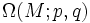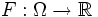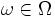Euler-Lagrange equation of a functional

(Redirected from Euler-Lagrange equation)
Let$M$ be a differential manifold and$\Omega(M;p,q)$ be the path space viz the space of piecewise smooth paths from$p$ to$q$ in$M$. Let$F:\Omega \to \R$ be a functional.
The Euler-Lagrange equation is the equation that$\omega \in \Omega$ needs to satisfy to be a critical path of$F$, viz, the equation it must satisfy so that for every piecewise smooth variation$W$ of$\omega$, the derivative of$F$ along$W$ is zero.